International
Tables for
Crystallography
Volume D
Physical properties of crystals
Edited by A. Authier

International Tables for Crystallography (2006). Vol. D, ch. 1.1, pp. 6-7

## Section 1.1.2.4.3. Properties of the metric tensor

A. Authiera*

aInstitut de Minéralogie et de la Physique des Milieux Condensés, Bâtiment 7, 140 rue de Lourmel, 75015 Paris, France
Correspondence e-mail: aauthier@wanadoo.fr

#### 1.1.2.4.3. Properties of the metric tensor

| top | pdf |

In a change of basis, following (1.1.2.3)and (1.1.2.5), the's transform according to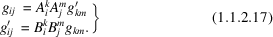Let us now consider the scalar products,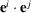, of two contravariant basis vectors. Using (1.1.2.11)and (1.1.2.13), it can be shown that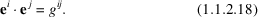In a change of basis, following (1.1.2.16), the's transform according to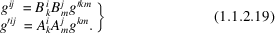The volumes V ′ and V of the cells built on the basis vectors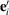and, respectively, are given by the triple scalar products of these two sets of basis vectors and are related by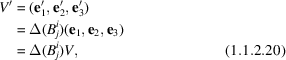where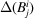is the determinant associated with the transformation matrix between the two bases. From (1.1.2.17)and (1.1.2.20), we can write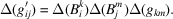If the basisis orthonormal,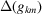and V are equal to one,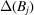is equal to the volume V ′ of the cell built on the basis vectorsand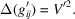This relation is actually general and one can remove the prime index: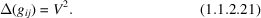In the same way, we have for the corresponding reciprocal basis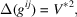whereis the volume of the reciprocal cell. Since the tables of the's and of the's are inverse, so are their determinants, and therefore the volumes of the unit cells of the direct and reciprocal spaces are also inverse, which is a very well known result in crystallography.